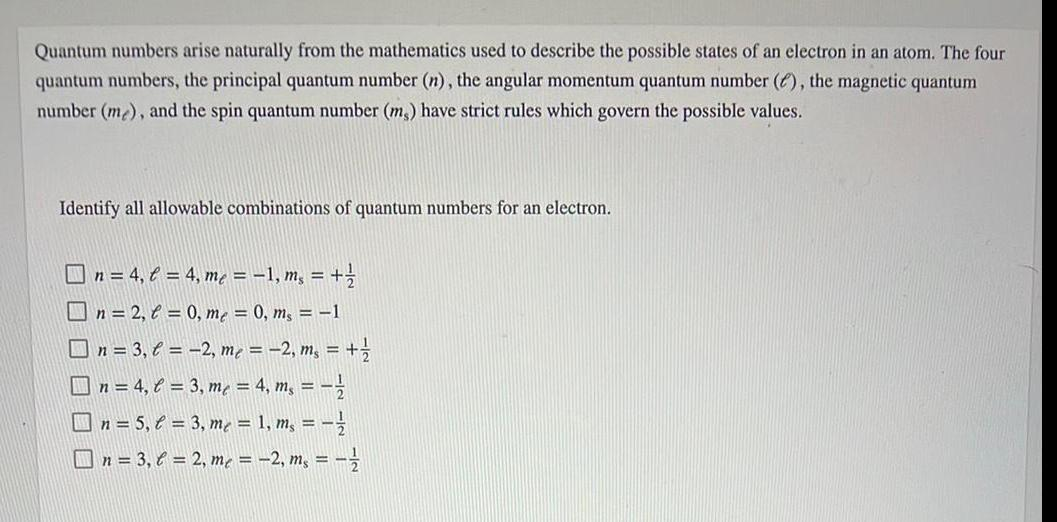Organic Chemistry
Isomerism
Quantum numbers arise naturally from the mathematics used to describe the possible states of an electron in an atom. The four quantum numbers, the principal quantum number (n), the angular momentum quantum number (), the magnetic quantum number (me), and the spin quantum number (ms) have strict rules which govern the possible values. Identify all allowable combinations of quantum numbers for an electron. n = 4₁ l = 4, m₁ = − 1, m, = + 1/ n=2, = 0, me = 0, ms = 1 n = 3, l = -2, me = -2, ms = + 1/2 n = 4, C = 3, m² = 4, m₁ = = ²/ n = 5₁ € = 3, me = 1, ms == // n = 3,6 = 2, me = -2, ms = 1/2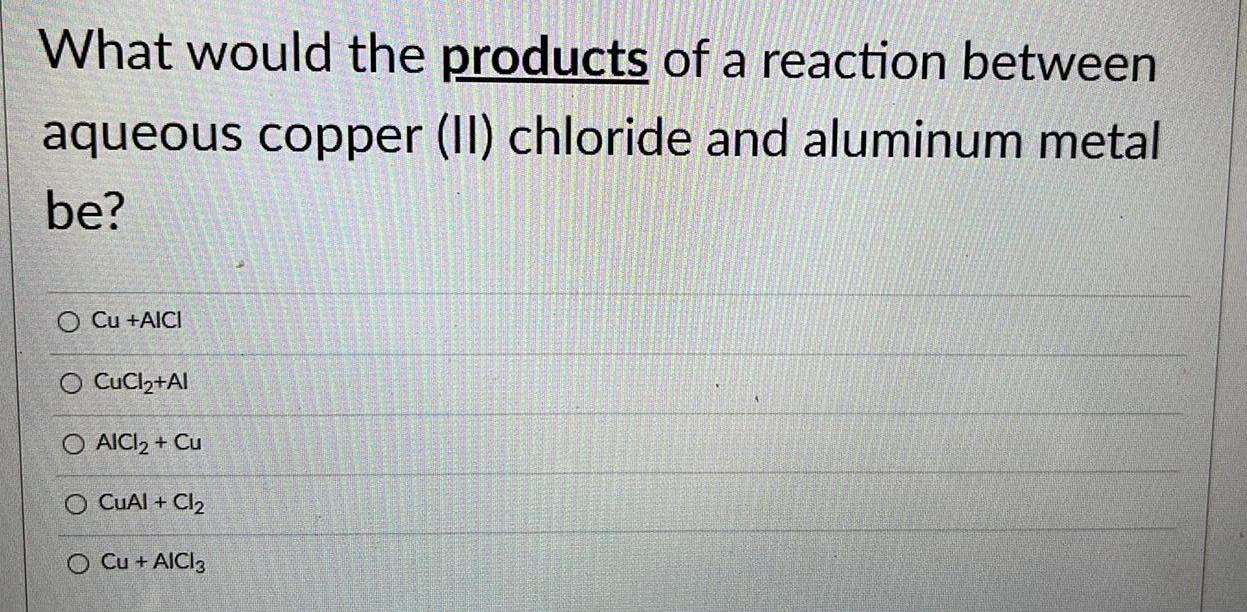Organic Chemistry
Isomerism
What would the products of a reaction between aqueous copper (II) chloride and aluminum metal be? Cu +AICI CuCl2+Al AICI₂ + Cu CuAl + Cl₂ Cu + AICI 3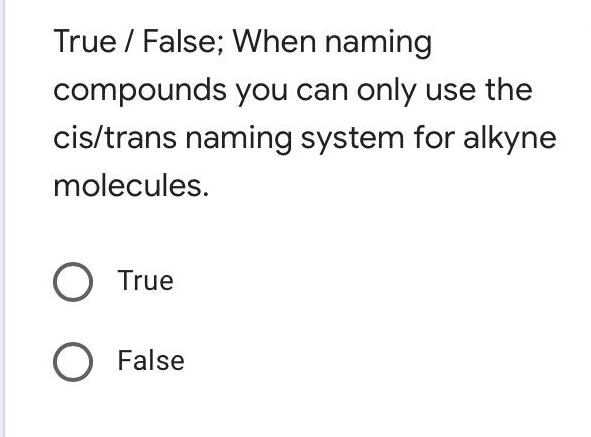Organic Chemistry
Isomerism
True / False; When naming compounds you can only use the cis/trans naming system for alkyne molecules. True False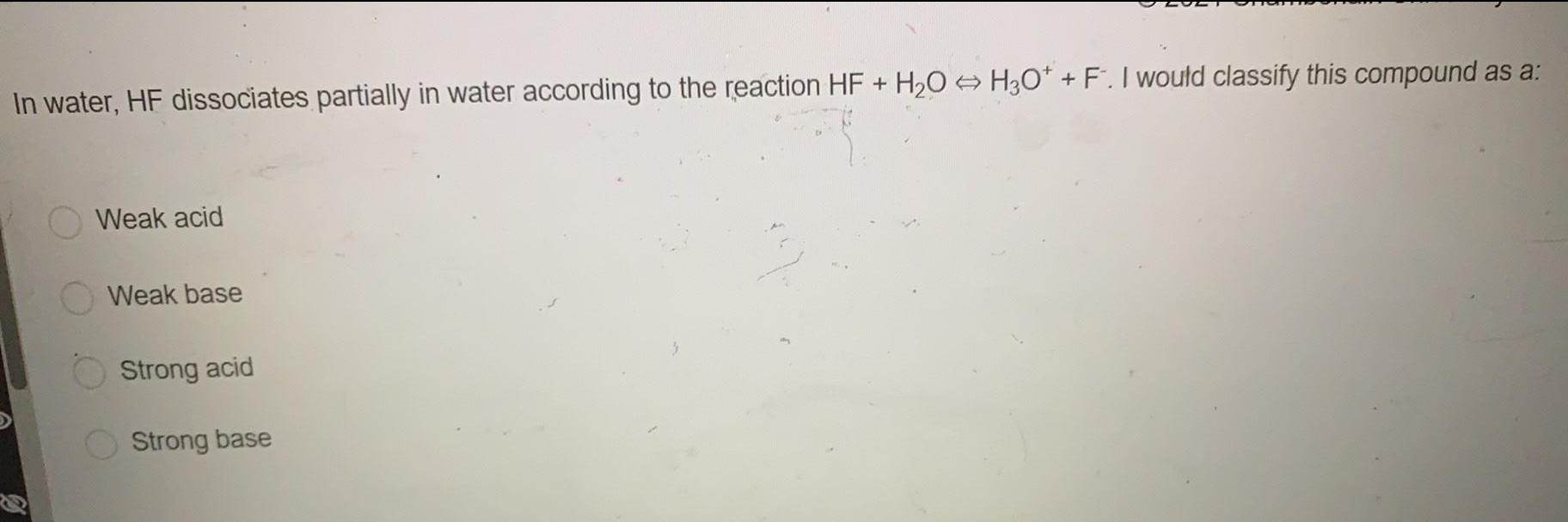Organic Chemistry
Isomerism
In water, HF dissociates partially in water according to the reaction HF + H₂O → H3O* + F. I would classify this compound as a: Weak acid Weak base Strong acid Strong base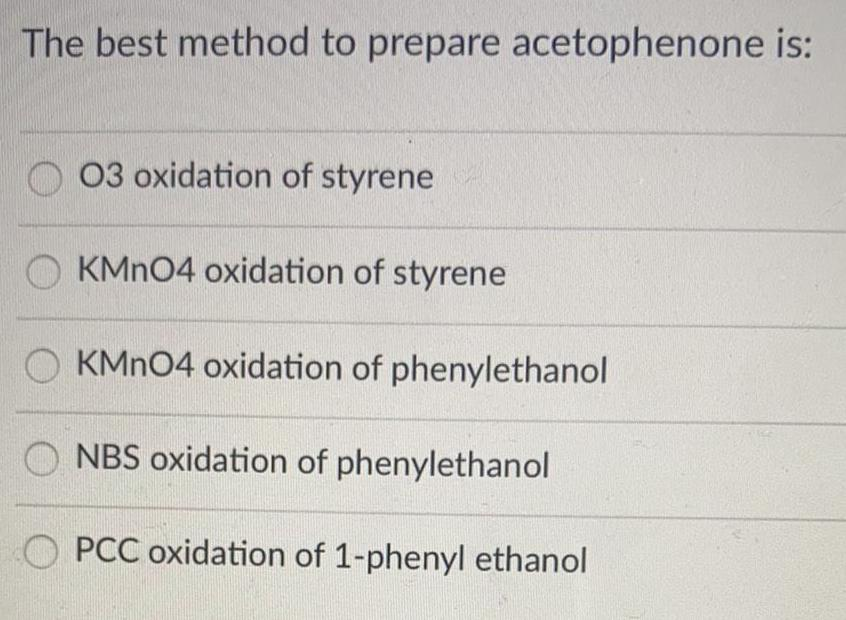Organic Chemistry
Isomerism
The best method to prepare acetophenone is: O3 oxidation of styrene KMnO4 oxidation of styrene KMnO4 oxidation of phenylethanol NBS oxidation of phenylethanol PCC oxidation of 1-phenyl ethanol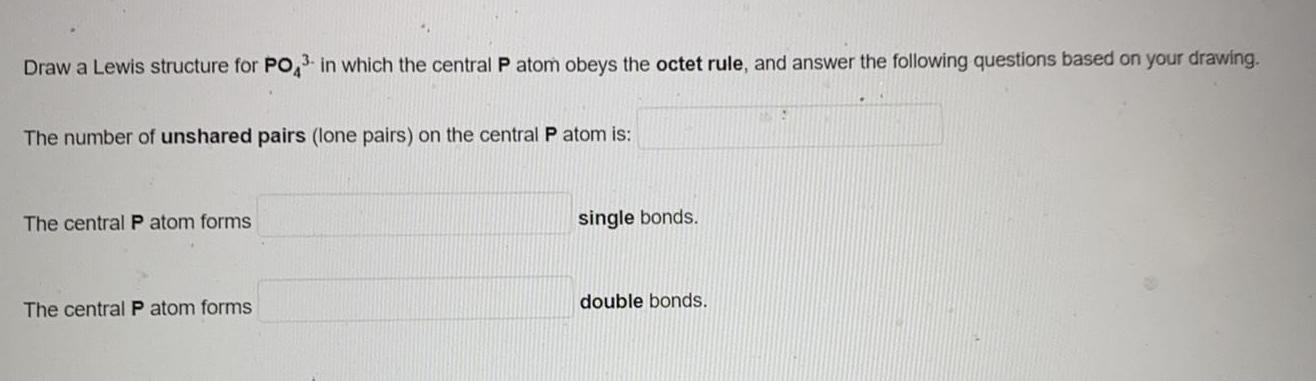Organic Chemistry
Isomerism
Draw a Lewis structure for PO,³ in which the central P atom obeys the octet rule, and answer the following questions based on your drawing. The number of unshared pairs (lone pairs) on the central P atom is: The central P atom forms _ single bonds. The central P atom forms _double bonds.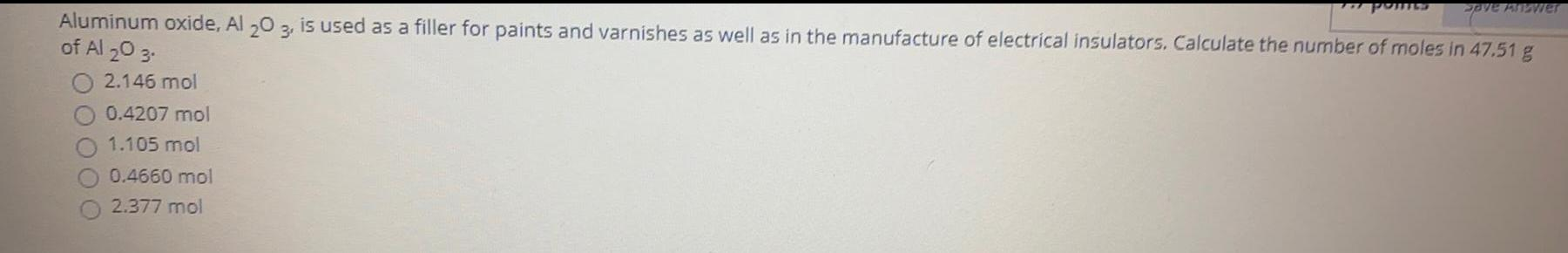Organic Chemistry
Isomerism
Aluminum oxide, Al 203, is used as a filler for paints and varnishes as well as in the manufacture of electrical insulators. Calculate the number of moles in 47.51 g of Al2O3. 2.146 mol 0.4207 mol 1.105 mol 0.4660 mol 2.377 mol Save Answer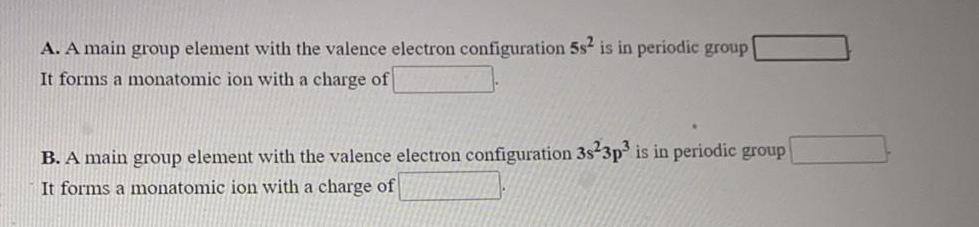Organic Chemistry
Isomerism
A. A main group element with the valence electron configuration 5s² is in periodic group It forms a monatomic ion with a charge of B. A main group element with the valence electron configuration 3s23p3 is in periodic group It forms a monatomic ion with a charge of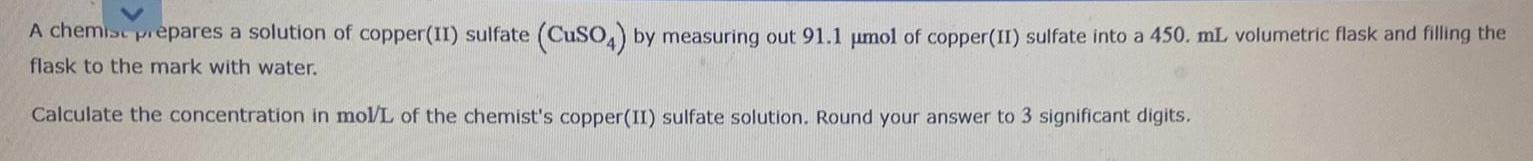Organic Chemistry
Isomerism
A chemise prepares a solution of copper(II) sulfate (CuSO4) by measuring out 91.1 μmol of copper (II) sulfate into a 450. mL. volumetric flask and filling the flask to the mark with water. Calculate the concentration in mol/L of the chemist's copper(II) sulfate solution. Round your answer to 3 significant digits.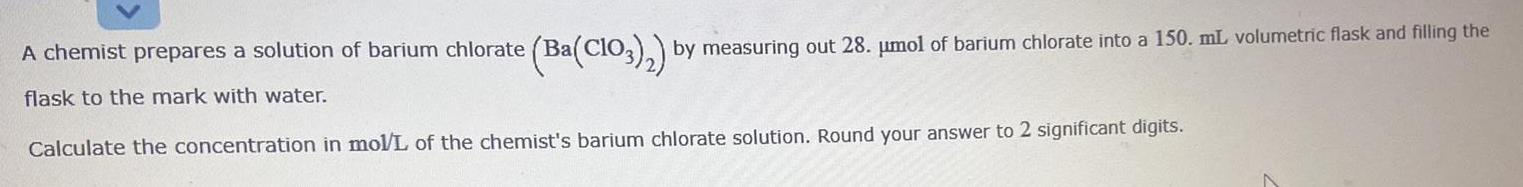Organic Chemistry
Isomerism
A chemist prepares a solution of barium chlorate (Ba(ClO3)₂) by measuring out 28. µmol of barium chlorate into a 150. mL volumetric flask and filling the flask to the mark with water. Calculate the concentration in mol/L of the chemist's barium chlorate solution. Round your answer to 2 significant digits.Organic Chemistry
Isomerism
Complete the Lewis structure for this species: CO2 Enter the total number of valence electrons in the box. Valence electrons: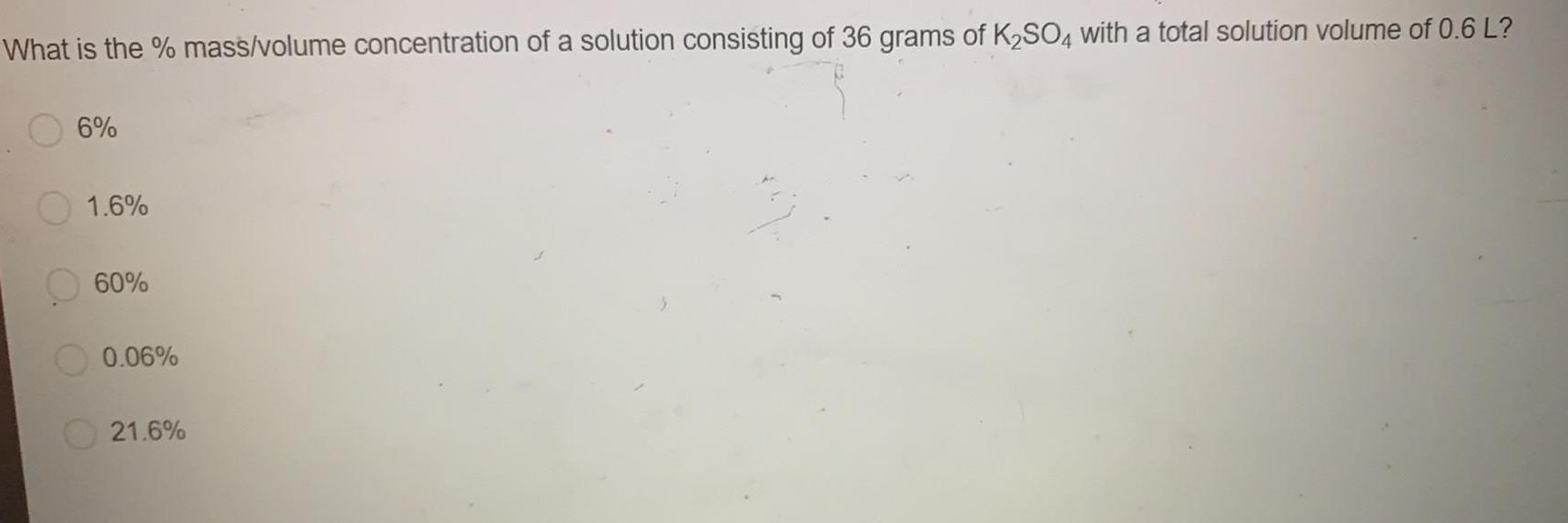Organic Chemistry
Isomerism
What is the % mass/volume concentration of a solution consisting of 36 grams of K₂SO4 with a total solution volume of 0.6 L? 6% 1.6% 60% 0.06% 21.6%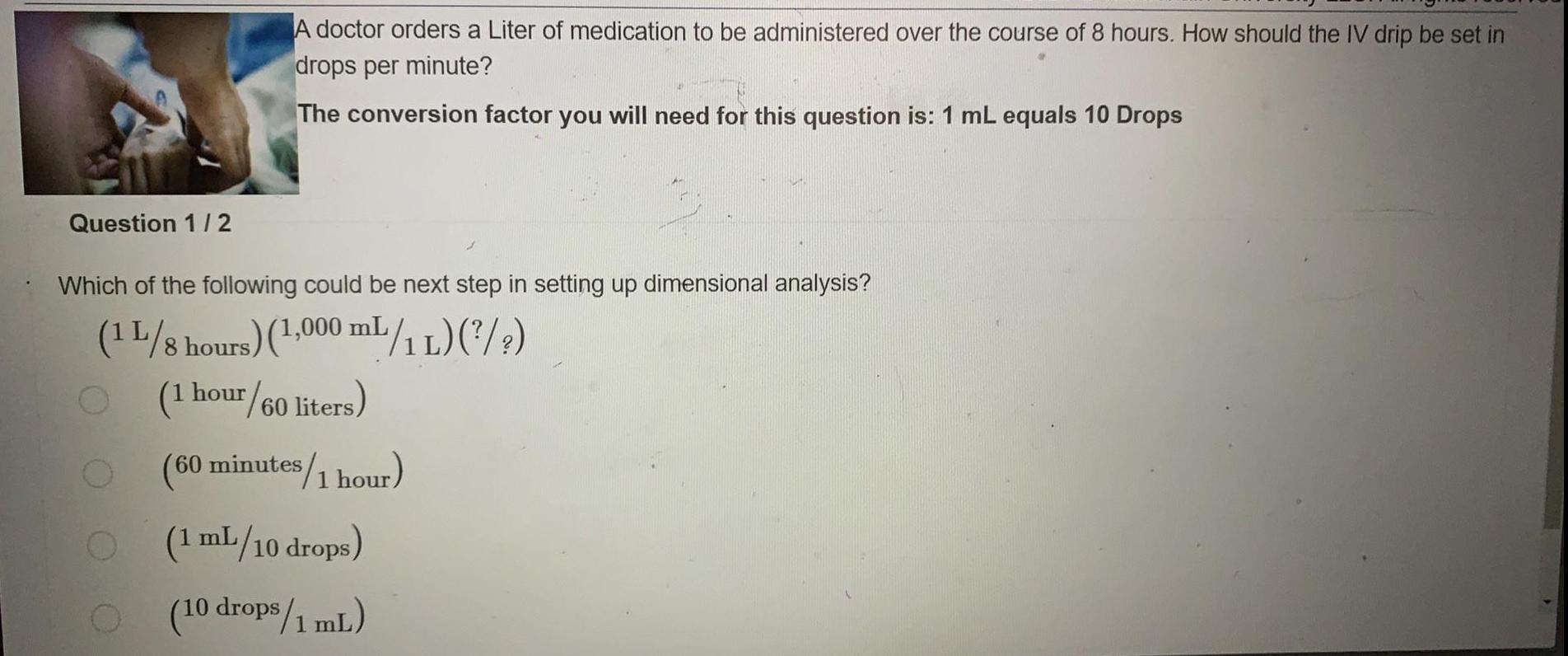Organic Chemistry
Isomerism
A doctor orders a Liter of medication to be administered over the course of 8 hours. How should the IV drip be set in drops per minute? The conversion factor you will need for this question is: 1 mL equals 10 Drops Which of the following could be next step in setting up dimensional analysis? (1L/8 hours) (1,000 mL/1L) (?/?) (1 hour/60 liters) (60 minutes/1 hour) (1 mL/10 drops) (10 drops/1 mL)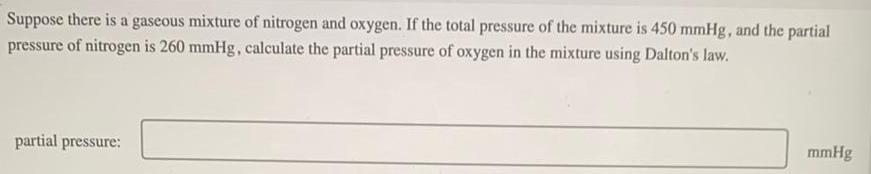Organic Chemistry
Isomerism
Suppose there is a gaseous mixture of nitrogen and oxygen. If the total pressure of the mixture is 450 mmHg, and the partial pressure of nitrogen is 260 mmHg, calculate the partial pressure of oxygen in the mixture using Dalton's law. partial pressure: mmHg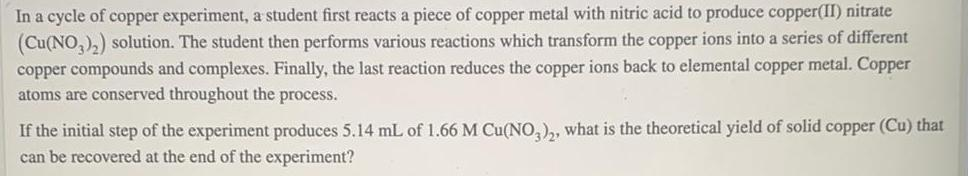Organic Chemistry
Isomerism
In a cycle of copper experiment, a student first reacts a piece of copper metal with nitric acid to produce copper(II) nitrate (Cu(NO3)₂) solution. The student then performs various reactions which transform the copper ions into a series of different copper compounds and complexes. Finally, the last reaction reduces the copper ions back to elemental copper metal. Copper atoms are conserved throughout the process. If the initial step of the experiment produces 5.14 mL of 1.66 M Cu(NO3)2, what is the theoretical yield of solid copper (Cu) that can be recovered at the end of the experiment?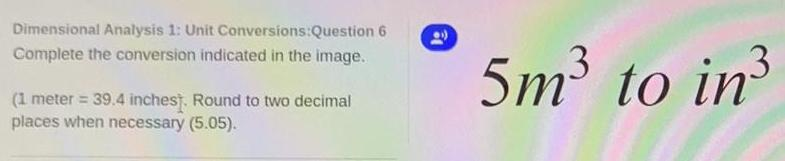Organic Chemistry
Isomerism
Dimensional Analysis 1: Unit Conversions:Question 6 Complete the conversion indicated in the image. (1 meter = 39.4 inches). Round to two decimal places when necessary (5.05).